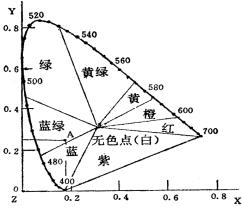# 色差仪的CIE1931 Yxy表色方法ρ= 物体表面的亮度/入射光源的亮度=Y / Y0Y=Y
x=X/（X+Y+Z）
y=Y/（X+Y+Z）

X=xY/y
Y=Y （5-14）
Z=(1-x-y)Y/y

Pi5红包